Click to Chat

1800-1023-196

+91-120-4616500

CART 0

• 0

MY CART (5)

Use Coupon: CART20 and get 20% off on all online Study Material

ITEM
DETAILS
MRP
DISCOUNT
FINAL PRICE
Total Price: Rs.

There are no items in this cart.
Continue Shopping• Complete JEE Main/Advanced Course and Test Series
• OFFERED PRICE: Rs. 15,900
• View Details

```Chapter 3: Squares and Square Roots Exercise – 3.4

Question: 1

Write the possible unit’s digits of the square root of the following numbers. Which of these numbers are odd square roots?

(i) 9801

(ii) 99856

(iii) 998001

(iv) 657666025

Solution:

(i) The unit digit of the number 9801 is 1. So, the possible unit digits are 1 or 9. Note that 9801 is equal to 992. Hence, the square root is an odd number.

(ii) The unit digit of the number 99856 is 6. So, the possible unit digits are 4 or 6 (Table 3.4). Since its last digit is 6 (an even number), it cannot have an odd number as its square root.

(iii) The unit digit of the number 998001 is 1. So, the possible unit digits are 1 or 9. Note that 998001 is equal to (33 × 37)2. Hence, the square root is an odd number.

(iv) The unit digit of the number 657666025 is 5. So, the only possible unit digit is 5. Note that 657666025 is equal to (5 × 23 × 223)2. Hence, the square root is an odd number.

Hence, among the given numbers, (i), (iii) and (iv) have odd numbers as their square roots.

Question: 2

Find the square root of each of the following by prime factorization:

(i) 441

(ii) 196

(iii) 529

(iv) 1764

(v) 1156

(vi) 4096

(vii) 7056

(viii) 8281

(ix) 11664

(x) 47089

(xi) 24336

(xii) 190969

(xiii) 586756

(xiv) 27225

(xv) 3013696

Solution:

(i) Resolving 441 into prime factors:

441= 3 x 3 x 7 x 7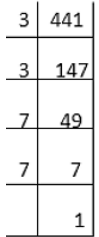Grouping the factors into pairs of equal factors:

441 = (3 x 3) x (7 x 7)

Taking one factor for each pair, we get the square root of 441:

3 x 7 = 21

(ii) Resolving 196 into prime factors:

196 = 2 x 2 x 7 x 7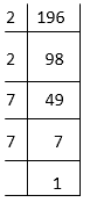Grouping the factors into pairs of equal factors:

196 = (2 x 2) x (7 x 7)

Taking one factor for each pair, we get the square root of 196:

2 × 7 = 14

(iii) Resolving 529 into prime factors:

529 = 23 x 23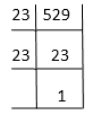Grouping the factors into pairs of equal factors:

529 = (23 x 23)

Taking one factor for each pair, we get the square root of 529 as 23.

(iv) Resolving 1764 into prime factors:

1764 = 2 x 2 x 3 x 3 x 7 x 7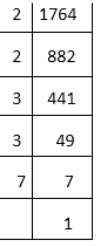Grouping the factors into pairs of equal factors:

1764 = (2 x 2) x (3 x 3) x (7 x 7)

Taking one factor for each pair, we get the square root of 1764:

2 x 3 x 7 = 42

(v) Resolving 1156 into prime factors:

1156 = 2 x 2 x 17 x 17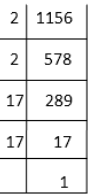Grouping the factors into pairs of equal factors:

1156 = (2 × 2) × (17 × 17)

Taking one factor for each pair, we get the square root of 1156:

2 × 17 = 34

(vi) Resolving 4096 into prime factors:

4096 = (2 x 2) x (2 x 2) x (2 x 2) x (2 x 2) x (2 x 2) x (2 x 2)Grouping the factors into pairs of equal factors:

4096 = (2 x 2) x (2 x 2) x (2 x 2) x (2 x 2) x (2 x 2) x (2 x 2)

Taking one factor for each pair, we get the square root of 4096:

(2 x 2) x (2 x 2) x (2 x 2) = 64

(vii) Resolving 7056 into prime factors:

7056 = (2 x 2) x (2 x 2) x (3 x 3) x (7 x 7)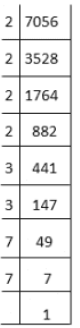Grouping the factors into pairs of equal factors:

7056 = (2 × 2) × (2 × 2) × (3 × 3) × (7 × 7)

Taking one factor for each pair, we get the square root of 7056:

2 × 2 × 3 × 7 = 84

(viii) Resolving 8281 into prime factors:

8281 = 7 × 7 × 13 × 13

Grouping the factors into pairs of equal factors:

8281 = (7 × 7) × (13 × 13)

Taking one factor for each pair, we get the square root of 8281:

7 × 13 = 91

(ix) Resolving 11664 into prime factors:

11664 = 2 × 2 × 2 × 2 × 3 × 3 × 3 × 3 × 3 × 3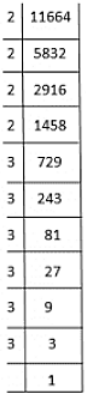Grouping the factors into pairs of equal factors:

11664 = (2 × 2) × (2 × 2) × (3 × 3) × (3 × 3) × (3 × 3)

Taking one factor for each pair, we get the square root of 11664:

2 × 2 × 3 × 3 × 3 = 108

(x) Resolving 47089 into prime factors:

47089 = 7 × 7 × 31 × 31Grouping the factors into pairs of equal factors:

47089 = (7 × 7) × (31 × 31)

Taking one factor for each pair, we get the square root of 47089:

7 × 31 = 217

(xi) Resolving 24336 into prime factors:

24336 = 2 × 2 × 2 × 2 × 3 × 3 × 13 × 13Grouping the factors into pairs of equal factors:

24336 = (2 × 2) × (2 × 2) × (3 × 3) × (13 × 13)

Taking one factor for each pair, we get the square root of 24336:

2 × 2 × 3 × 13 = 156

(xii) Resolving 190969 into prime factors:

190969 = 19 × 19 × 23 × 23

Grouping the factors into pairs of equal factors:

190969 = (19 × 19) × (23 × 23)

Taking one factor for each pair, we get the square root of 190969:

19 × 23 = 437

(xiii) Resolving 586756 into prime factors:

568756 = 2 × 2 × 383 × 383

Grouping the factors into pairs of equal factors:

568756 = (2 × 2) × (383 × 383)

Taking one factor for each pairs, we get the square root of 568756;

2 × 383 = 766

(xiv) Resolving 27225 into prime factors:

27225 = 3 × 3 × 5 × 5 × 11 × 11

Grouping the factors into pairs of equal factors:

27225 = (3 × 3) × (5 × 5) × (11 × 11)

Taking one factor for each pair, we get the square root of 27225:

3 × 5 × 11 = 165

(xv) Resolving 3013696 into prime factors:

3013696 = 2 × 2 × 2 × 2 × 2 × 2 × 7 × 7 × 31 × 31Grouping the factors into pairs of equal factors:

3013696 = (2 × 2) × (2 × 2) × (2 × 2) × (7 × 7) × (31 × 31)

Taking one factor for each pairs, we get the square root of 3013696;

2 × 2 × 2 × 7 × 31 = 1736

Question: 3

Find the smallest number by which 180 must be multiplied so that it becomes a perfect square. Also, find the square root of the perfect square so obtained.

Solution:

The prime factorization of 180:

180 = 2 x 2 x 3 x 3 x 5

Grouping the factors into pairs of equal factors, we get:

180 = (2 x 2) x (3 x 3) x 5

The factor, 5 does not have a pair.

Therefore, we must multiply 180 by 5 to make a perfect square. The new number is:

(2 x 2) x (3 x 3) x (5 x 5) = 900

Taking one factor from each pair on the LHS, the square root of the new number is 2 x 3 x 5, which is equal to 30.

Question: 4

Find the smallest number by which 147 must be multiplied so that it becomes a perfect square. Also, find the square root of the number so obtained.

Solution:

The prime factorization of 147:

147 = 3 x 7 x 7

Grouping the factors into pairs of equal factors, we get:

147 = 3 x (7 x 7)

The factor, 3 does not have a pair. Therefore, we must multiply 147 by 3 to make a perfect square. The new number is:

(3 x 3) x (7 x 7) = 441

Taking one factor from each pair on the LHS, the square root of the new number is 3 x 7, which is equal to 21.

Question: 5

Find the smallest number by which 3645 must be divided so that it becomes a perfect square. Also, find the square root of the resulting number.

Solution:

The prime factorization of 3645:

3645 = 3 x 3 x 3 x 3 x 3 x 3 x 5

Grouping the factors into pairs of equal factors, we get:

3645 = (3 x 3) x (3 x 3) x (3 x 3) x 5

The factor, 5 does not have a pair. Therefore, we must divide 3645 by 5 to make a perfect square. The new number is:

(3 x 3) x (3 x 3) x (3 x 3) = 729

Taking one factor from each pair on the LHS, the square root of the new number is 3 x 3 x 3, which is equal to 27.

Question: 6

Find the smallest number by which 1152 must be divided so that it becomes a perfect square. Also, find the square root of the number so obtained.

Solution:

The prime factorization of 1152:

1152 = 2 x 2 x 2 x 2 x 2 x 2 x 2 x 3 x 3

Grouping the factors into pairs of equal factors, we get:

1152 = (2 x 2) x (2 x 2) x (2 x 2) x (3 x 3) x 2

The factor, 2, at the end, does not have a pair. Therefore, we must divide 1152 by 2 to make a perfect square. The new number is:

(2 x 2) x (2 x 2) x (2 x 2) x (3 x 3) = 576

Taking one factor from each pair on the LHS, the square root of the new number is 2 x 2 x 2 x 3, which is equal to 24.

Question: 7

The product of two numbers is 1296.  If one of the numbers is 16 times the other, find the numbers.

Solution:

Let the two numbers be a and b.

From the first statement, we have:

a x b = 1296

If one number is 16 times the other, then we have:

b = 16 x a.

Substituting this value in the first equation, we get:

a x (16 x a) = 1296

By simplifying both sides, we get:

a2 = 1296/16 = 81

Hence, a is the square root of 81, which is 9.

To find b, use equation b = 16 x a.

Since a = 9: b = 16 x 9 = 144

So, the two numbers satisfying the question are 9 and 144.

Question: 8

A welfare association collected Rs. 202500 as donation from the residents. If each paid as many rupees as there were residents, find the number of residents.

Solution:

Let R be the number of residents.

Let r be the money in rupees donated by each resident.

Total donation = R x r = 202500

Since the money received as donation is the same as the number of residents:

r = R.

Substituting this in the first equation, we get:

R x R = 202500, R2 = 202500

R2 = (2 x 2) x (5 x 5) x (5 x 5) x (3×3)2

R = 2 x 5 x 5 x 3 x 3 = 450

So, the number of residents is 450.

Question: 9

A society collected Rs. 92.16. Each member collected as many paise as there were members. How many members were there and how much did each contribute?

Solution:

Let M be the number of members.

Let r be the amount in paise donated by each member.

The total contribution can be expressed as follows:

M x r = Rs 92.16 = 9216 paise

Since the amount received as donation is the same as the number of members:

r = M

Substituting this in the first equation, we get:

M x M = 9216

M2 = 2 x 2 x 2 x 2 x 2 x 2 x 2 x 2 x 2 x 2  x 3 x 3

M2 = (2 x 2) x (2 x 2) x (2 x 2) x (2 x 2) x (2 x 2) x (3 x 3)

M = 2 x 2 x 2 x 2 x 2 x 3 = 96

To find r, we can use the relation r = M.

Let M be the number of members.

Let r be the amount in paise donated by each member.

The total contribution can be expressed as follows:

M x r = Rs 92.16 = 9216 paise

Since the amount received as donation is the same as the number of members:

r = 96

So, there are 96 members and each paid 96 paise.

Question: 10

A school collected Rs. 2304 as fees from its students. If each student paid as many paise as there were students in the school, how many students were there in the school?

Solution:

Let S be the number of students.

Let r be the money donated by each student.

The total contribution can be expressed by (s)(r) = Rs 2304

Since each student paid as many paise as the number of students, then r = S.

Substituting this in the first equation, we get:

S x S = 2304

S2= 2 x 2 x  2 x 2  x 2 x 2 x 2 x  2 x 3 x 3

S2 = (2 x 2) x (2 x 2) x (2 x 2) x (2 x 2) x (3 x 3)

S = 2 x 2 x 2 x 2 x 3 = 48

So, there are 48 students in total in the school.

Question: 11

The area of a square field is 5184 m2. A rectangular field, whose length is twice its breadth has its perimeter equal to the perimeter of the square field. Find the area of the rectangular field.

Solution:

First, we have to find the perimeter of the square.

The area of the square is r2, where r is the side of the square.

Then, we have the equation as follows:

r2 = 5184 = (2 x 2) x (2 x 2) x (2 x 2) x (3 x 3) x (3 x 3)

Taking the square root, we get r = 2 x 2 x 2 x 3 x 3 = 72

Hence the perimeter of the square is 4 x r = 288 m

Now, let L be the length of the rectangular field.

Let W be the width of the rectangular field.

The perimeter is equal to the perimeter of square.

Hence, we have:

2(L + W) = 288

Moreover, since the length is twice the width:

L = 2 x W.

Substituting this in the previous equation, we get:

2 x (2 x W+ W) = 288 3 x W= 144 W= 48

To find L: L = 2 x W = 2 x 48 = 96

Area of the rectangular field = L x W = 96 x 48 = 4608 m2

Question: 12

Find the least square number, exactly divisible by each one of the numbers:

(i) 6, 9, 15 and 20

(ii) 8, 12, 15 and 20

Solution:

(i) The smallest number divisible by 6, 9, 15 and 20 is their L.C.M., which is equal to 60.

Factorizing 60 into its prime factors:

60 = 2  x 2 x 3 x 5

Grouping them into pairs of equal factors:

60 = (2 x 2) x 3 x 5

The factors 3 and 5 are not paired.

To make 60 a perfect square, we have to multiply it by 3 x 5, i.e. by 15.

The perfect square is 60 x 15, which is equal to 900.

(ii) The smallest number divisible by 8, 12, 15 and 20 is their L.C.M., which is equal to 120.

Factorizing 120 into its prime factors:

120 =2 x 2 x 2 x 3 x 5

Grouping them into pairs of equal factors:

120 = (2 x 2) x 2 x 3 x 5

The factors 2, 3 and 5 are not paired.

To make 120 into a perfect square, we have to multiply it by 2 x 3 x 5, i.e. by 30.

The perfect square is 120 x 30, which is equal to 3600.

Question: 13

Find the square roots of 121 and 169 by the method of repeated subtraction.

Solution:

To find the square root of 121:

121 – 1 = 120

120 – 3 = 117

117 – 5 = 112

112 – 7 = 105

105 – 9 = 96

96 – 11 = 85

85 – 13 = 72

72 – 15 = 57

57 – 17 = 40

40 – 19 = 21

21 – 21 = 0

In total, there are 11 numbers to subtract from 121. Hence, the square root of 121 is 11.

To find the square root of 169:

169 – 1 = 168

168 – 3 = 165

165 – 5 = 160

160 – 7 = 153

153 – 9 = 144

144 – 11 = 133

133 – 13 = 120

120 – 15 = 105

105 – 17 = 88

88 – 19 = 69

69 – 21 = 48

48 – 23 = 25

25 – 25 = 0

In total, there are 13 numbers to subtract from 169. Hence, the square root of 169 is 13.

Question: 14

Write the prime factorization of the following numbers and hence find their square roots:

(i) 7744

(ii) 9604

(iii) 5929

(iv) 7056

Solution:

(i) The prime factorization of 7744:

7744 = 2 × 2 × 2 × 2 × 2 × 2 × 11 × 11

Grouping them into one pair of equal factors, we get:

7744 = (2 × 2) × (2 × 2) × (2 × 2) × (11 × 11)

Taking one factor from each pairs, we will get:

√(7744) = 2 × 2 × 2 × 11 = 88

(ii) The prime factorization of 9604:

9604 = 2 × 2 × 7 × 7 × 7 × 7

Grouping them into one pair of equal factors, we get:

9604 = (2 × 2) × (7 × 7) × (7 × 7)

Taking one factor from each pairs, we will get:

√(9604) = 2 × 7 × 7 = 98

(iii) The prime factorization of 5929:

5929 = 7 × 7 × 11 × 11

Grouping them into one pair of equal factors, we get:

5929 = (7 × 7) × (11 × 11)

Taking one factor from each pairs, we will get:

√(5929)= 7 × 11 = 77

(iv) The prime factorization of 7056:

7056 = 2 × 2 × 2 × 2 × 3 × 3 × 7 × 7

Grouping them into one pair of equal factors, we get:

7056 = (2 × 2) × (2 × 2) × (3 × 3) × (7 × 7)

Taking one factor from each pairs, we will get:

√(7056) = 2 × 2 × 3 × 7 = 84

Question: 15

The student of class VIII of a school donated Rs. 2401 for PM’s National Relief Fund. Each student denoted as many rupees as the number of students in the class. Find the number of students in the class.

Solution:

Let S be the number of students.

Let r be the amount in rupees denoted by each student.

The total donation can be expressed by:

S × r = Rs. 2401

Since, the total amount in rupees is equal to the number of students

So, r is equal to S.

Substituting this in the first equation:

S × S = 2401

S2 = (7 × 7) × (7 × 7)

S = 7 × 7 = 49

So, there are 49 students in the class.

Question: 16

APT teacher wants to arrange maximum possible number of 6000 students in a field such that the number of rows is equal to the number of columns. Find the number of rows if 71 were left out after arrangement.

Solution:

Since, 71 students were left out, there are only 5929, i.e., (6000 – 72) students remaining.

Hence, the number of rows and columns is simply the square root of 5929.

Factorizing 5929 into its prime factors:

5929 = 7 × 7 × 11 × 11

Grouping them into pairs of equal factors:

5929 = (7 × 7) × (11 × 11)

The square root of 5929 = √(5929) = 7 × 11 = 77

Hence, in the arrangement, there were 77 rows of students.
```### Course Features

• 728 Video Lectures
• Revision Notes
• Previous Year Papers
• Mind Map
• Study Planner
• NCERT Solutions
• Discussion Forum
• Test paper with Video Solution